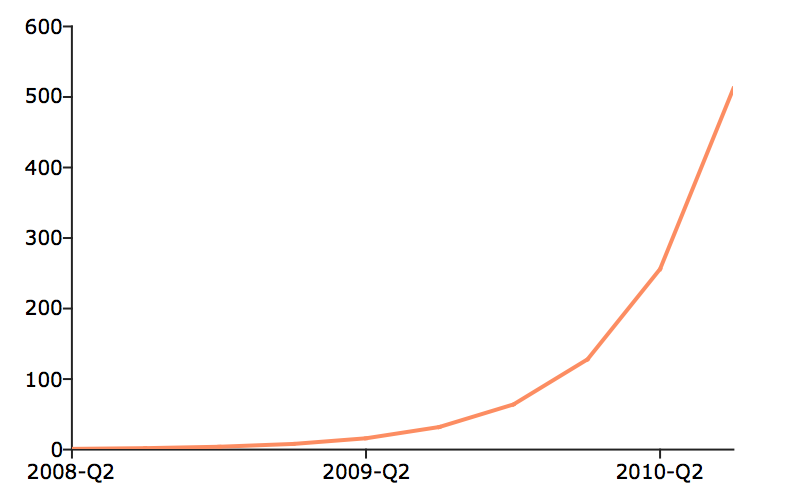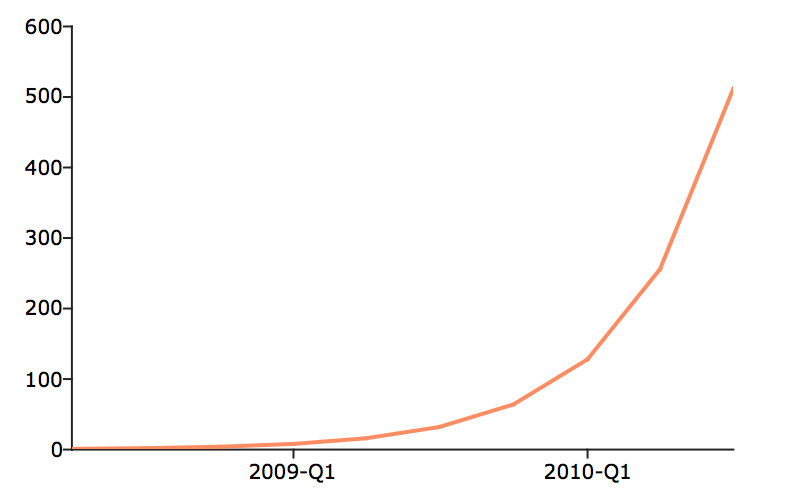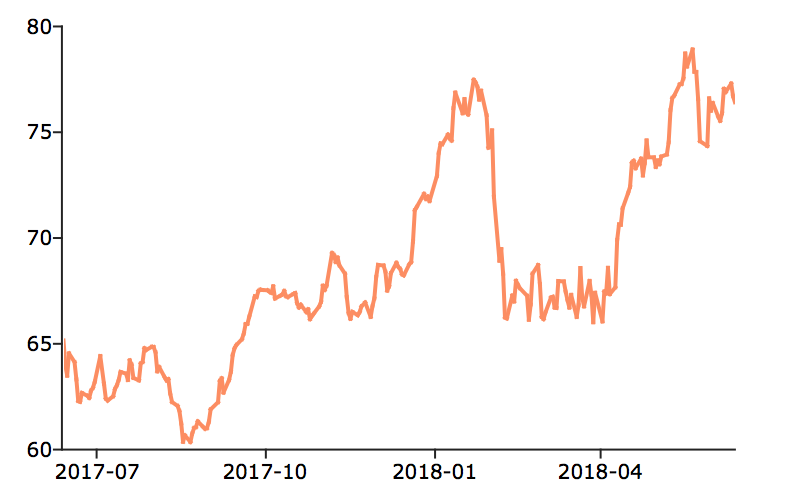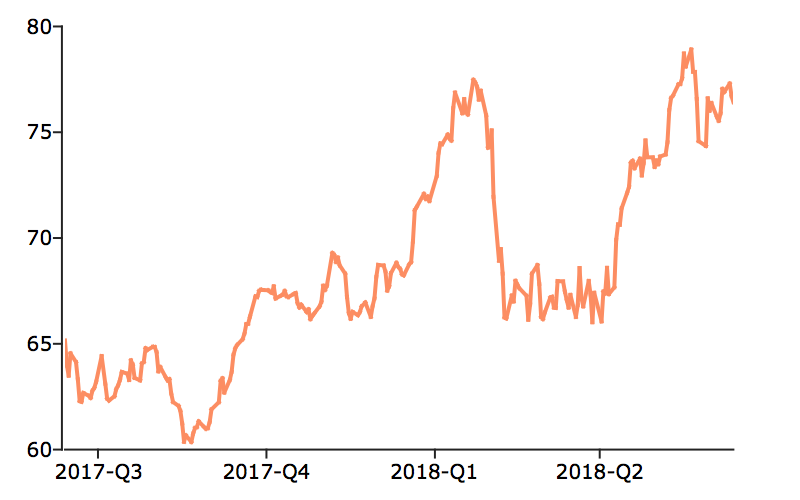# plotSetXTicInterval¶

## Purpose¶

Controls the interval between X-axis tick labels and also allows the user to specify the first tick to be labeled for 2-D graphs.

## Format¶

plotSetXTicInterval(&myPlot, ticInterval[, firstLabeled])
Parameters: &myPlot (struct pointer) – A plotControl structure pointer. ticInterval (scalar) – the distance between X-axis tick labels. firstLabeled (scalar) – Optional input, the value of the first X value on which to place a tick label.

## Examples¶

### XY plot¶

// Create the sequence 0.25, 0.5, 0.75...3
x = seqa(0.25, 0.25, 12);
y = sin(x);

// Declare plotControl structure
// and fill with default settings for XY plots
struct plotControl myPlot;
myPlot = plotGetDefaults("xy");

// Place the first X-tick label at 0.5
// and place additional ticks every 0.25 after
plotSetXTicInterval(&myPlot, 0.25, 0.5);

// Draw plot with applied X-tick settings
plotXY(myPlot, x, y);


### Scalar starting date¶// Declare and initialize plotControl structure
struct plotControl myPlot;
myPlot = plotGetDefaults("xy");

// Place one tick label every 4 x-values
ticInterval = 4;
plotSetXTicInterval(&myPlot, ticInterval);

// Start the time series in April of 2008
dtstart = 200804;

// Specify quarterly data
frequency = 4;

// Create the multiplicative sequence 1, 2, 4, 8...
y = seqm(1, 2, 10);

// Create a time series plot of the data.
plotTS(myPlot, dtstart, frequency, y);


If you would like to change the tick labels so that they start on the first full year, 2009, continuing with the example from above, execute the following lines:

// Set the optional 'firstLabeled' parameter
plotSetXTicInterval(&myPlot, ticInterval, 2009);
plotTS(myPlot, dtstart, frequency, y);


This new plot should now have tick labels only on the first quarters of each year:### Daily data with full time vector¶

// Fully pathed file name
fname = getGAUSSHome() \$+ "examples/xle_daily.xlsx";

// Load all observations from variables,

// Separate the 'date' vector and 'adjusted close'
// into different vectors
date_vec = data[., 1];
closing_price = data[., 2];

// Declare 'myPlot' to be a plotControl structure
// and fill with default settings for XY plots
struct plotControl myPlot;
myPlot = plotGetDefaults("xy");

// Draw the first X-tick label at July 2017
// Draw a new X-tick label every 3 label_units,
// which is 'months' in this case
plotSetXTicInterval(&myPlot, 3, 201707);

label_unit = "months";

// Create a time series plot of the data.
plotTS(myPlot, date_vec, label_unit, closing_price);Let’s keep the tick labels on the same locations, however, create 1 tick label every quarter, instead of every 3 months. The following code will accomplish this.

// Draw the first X-tick label at July 2017
// Draw a new X-tick label every 1 label_unit,
// which is 'quarters' in this case
plotSetXTicInterval(&myPlot, 1, 201707);

label_unit = "quarters";

// Create a time series plot of the data.
plotTS(myPlot, date_vec, label_unit, closing_price);## Remarks¶

plotSetXTicInterval() is supported for use with XY, Scatter, Contour and time series plots. It is ignored by other plot types.

This function sets an attribute in a plotControl structure. It does not affect an existing graph, or a new graph drawn using the default settings that are accessible from the Tools > Preferences > Graphics menu. See the GAUSS Graphics chapter for more information on the methods available for customizing your graphs.